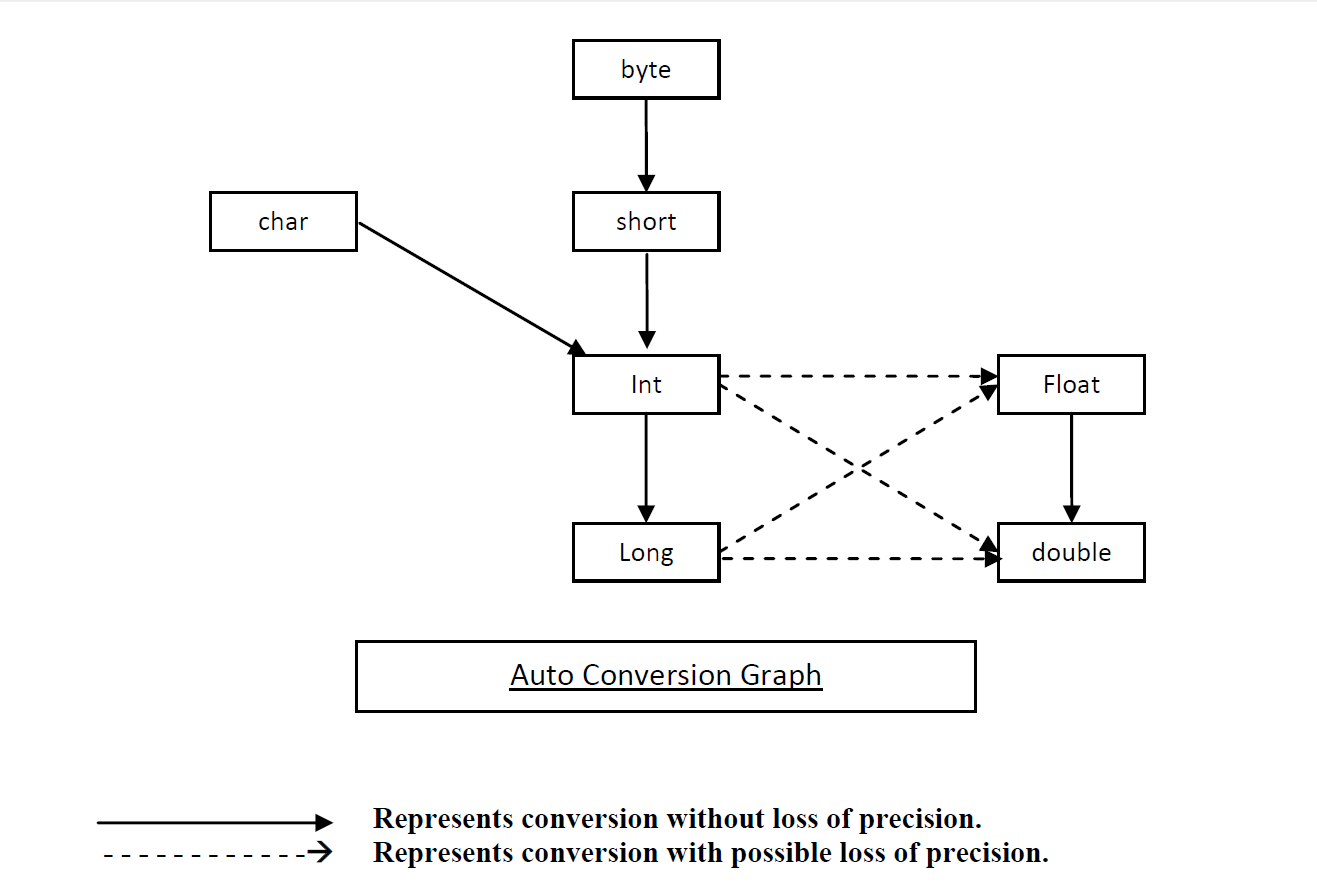• www.uptuexam.com
• GBTU Results 2014
• MTU Results 2014
• UPTU Circulars
• COP Result 2012,13,14
• UPTU Previous Papers
• GATE study material
• Robotics Projects
Thank you for visiting www.UPTUexam.com " Free download Exam materials, previous year question papers, free ebooks & Providing Important Questions To Do their Exams Well and Totally a FREE SERVICE for all engineering streams (B.Tech)."

### Type Casting / Type Conversion

##### Type Casting / Type Conversion

Converting the values of one type to another for the evaluation of an expression is called type casting or type conversion.

Type casting is of two types:-

1) Upcasting – Conversion of lower types to upper types is called upcasting. Upcasting is implicitly performed.

Upcasting is performed according to the following graph:-Explanation -
a.      Let n be an integer and n = 2n then to store n in memory in integer representation k bits are required.

For e.g
32768 = 215
0 111111111111111

15 bits are required to store 32768.

b.      In floating point representation number is not stored rather its exponent is stored.
i.e
32768 = 215
Then only 15 need to be stored.

n=2k and k = 2l where l<k then to store n only l bits are required.

2) Downcasting – Conversion of higher types to lower type is called downcasting. Downcasting need to be explicitly performed.

Syntax –
(target type) expression;

Example- float f = 12.45;    ==========>  float f = (float)12.45;  OR  float f = 12.45f;
By this statement compiler

1. PROGRAM – Rectangle.java
2. public class Rectangle
3. {
4. private int l, b;
5. public void Rectangle(int x, int y)
6. {
7. l = x;
8. b = y;
9. }
10. public void display()
11. {
12. System.out.println("Length = " + l);
13. System.out.println("Breadth = " + b);
14. }
15. public int area()
16. {
17. return l * b;
18. }
19. public void setDimension(int x, int y)
20. {
21. l = x;
22. b = y;
23. }
24. }
25. Syntax of creating object of a class-
26. className ReferenceVariable = new className();
27. OR
28. className ReferenceVariable;
29. ReferenceVariable = new className();

Reference Variable – is an implicit pointer that contains the reference of a class object. In Java, objects are dynamically created. Hence, they don’t have names. They are referred using the name of their reference variable.

In C++ :-
Rectangle r;Traditionally in languages when we write statement
int a=5, b=6, c=10;
a table is created during compilation.

 Variable Name Type Address Value Scope a int 0 + 1000 5 main B int 2 + 1000 6 main c int 4 + 1000 10 main

int * p;Address & Reference denotes two different things.
o Address represents actual memory location whereas Reference represents a mechanism of finding out objects.
 Different JRE provides different implementations of references.
o In Microsft JRE, References are implemented as pointers that is they actually holds the address of object.o In Sun Microsystem JRE, Reference Variables are implemented as pointer to pointer.In this implementation, Reference and Address are different.Now we will write a program RectTest.java to test the program Rectangle.java written above.

1. public class RectTest
2. {
3. public static void main(String[] args)
4. {
5. Rectangle r = new Rectangle();
6. r.l = 5;
7. r.b = 7;
8. r.setDimension(5, 4);
9. System.out.println("Dimension of rectangle r ");
10. r.display();
11. System.out.println("Area of r = " + r.area());
12. }
13. }

Output –Since variables l and b are declared private so we cannot access it from outside.

Now the same program RectTest.java again.

1. PROGRAM RectTest.java
2. public class RectTest
3. {
4. public static void main(String[] args)
5. {
6. Rectangle r = new Rectangle();
7. //r.l = 5; // Invalid statement
8. //r.b = 7; // Invalid statement
9. r.setDimension(5, 4);
10. System.out.println("Dimension of rectangle r ");
11. r.display();
12. System.out.println("Area of r = " + r.area());
13. }
14. }

OUTPUT –